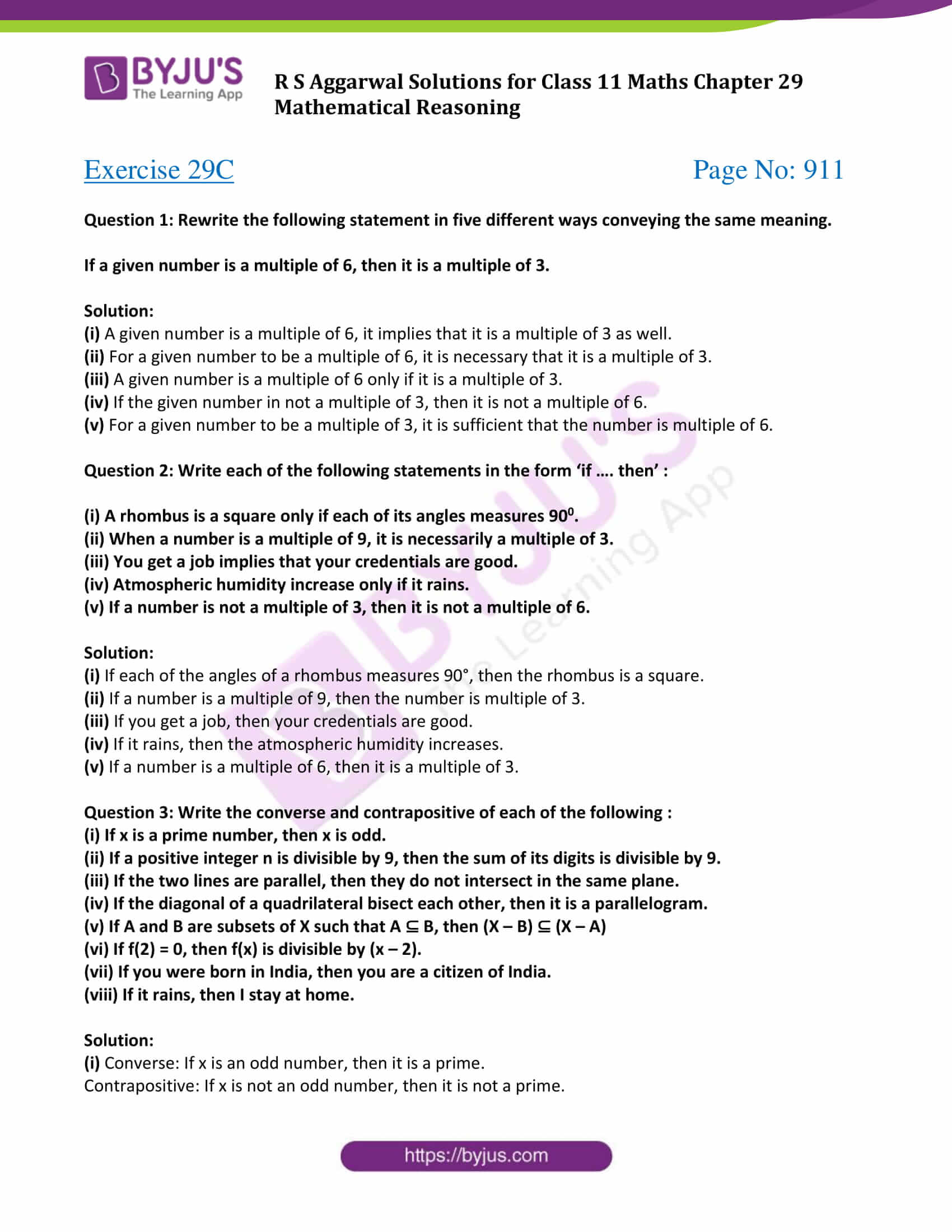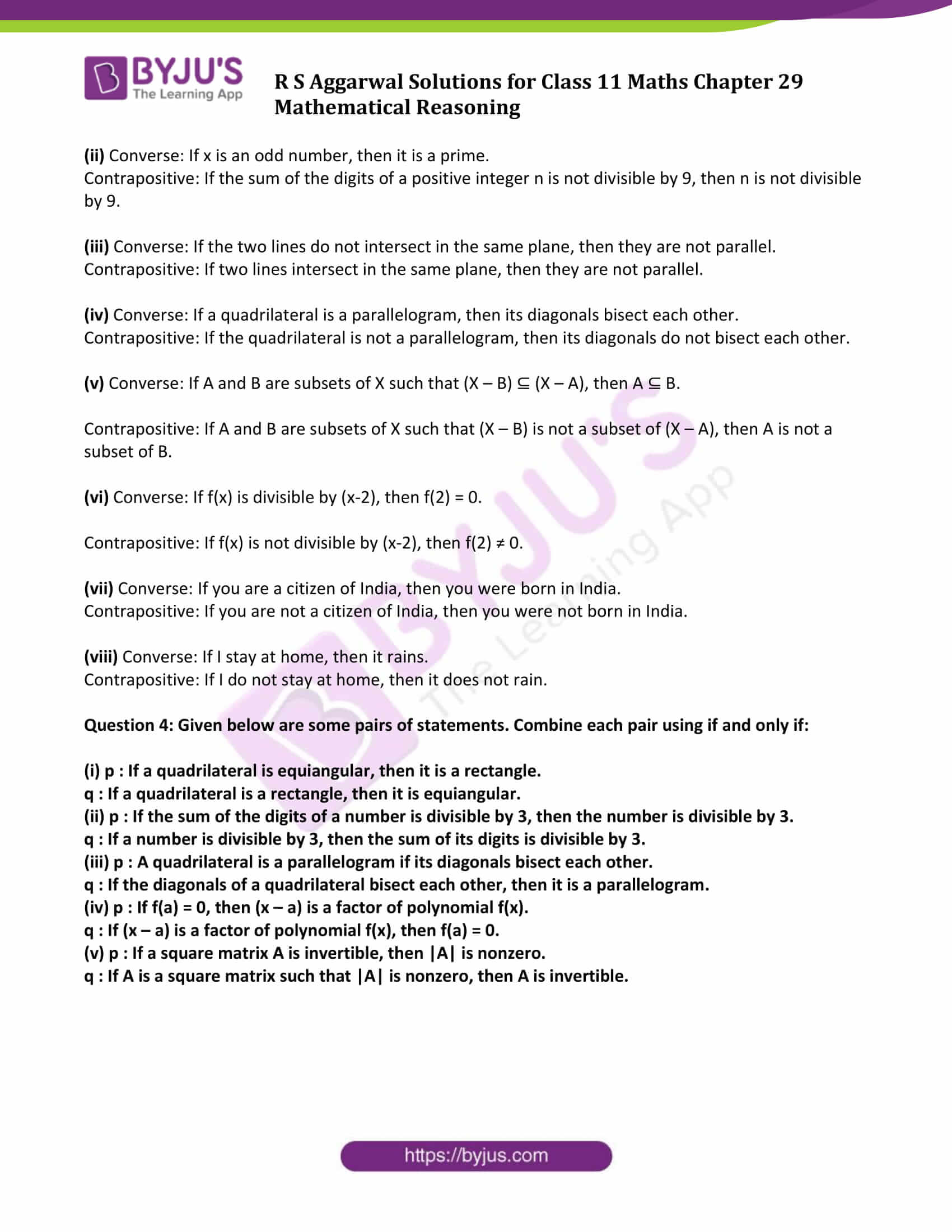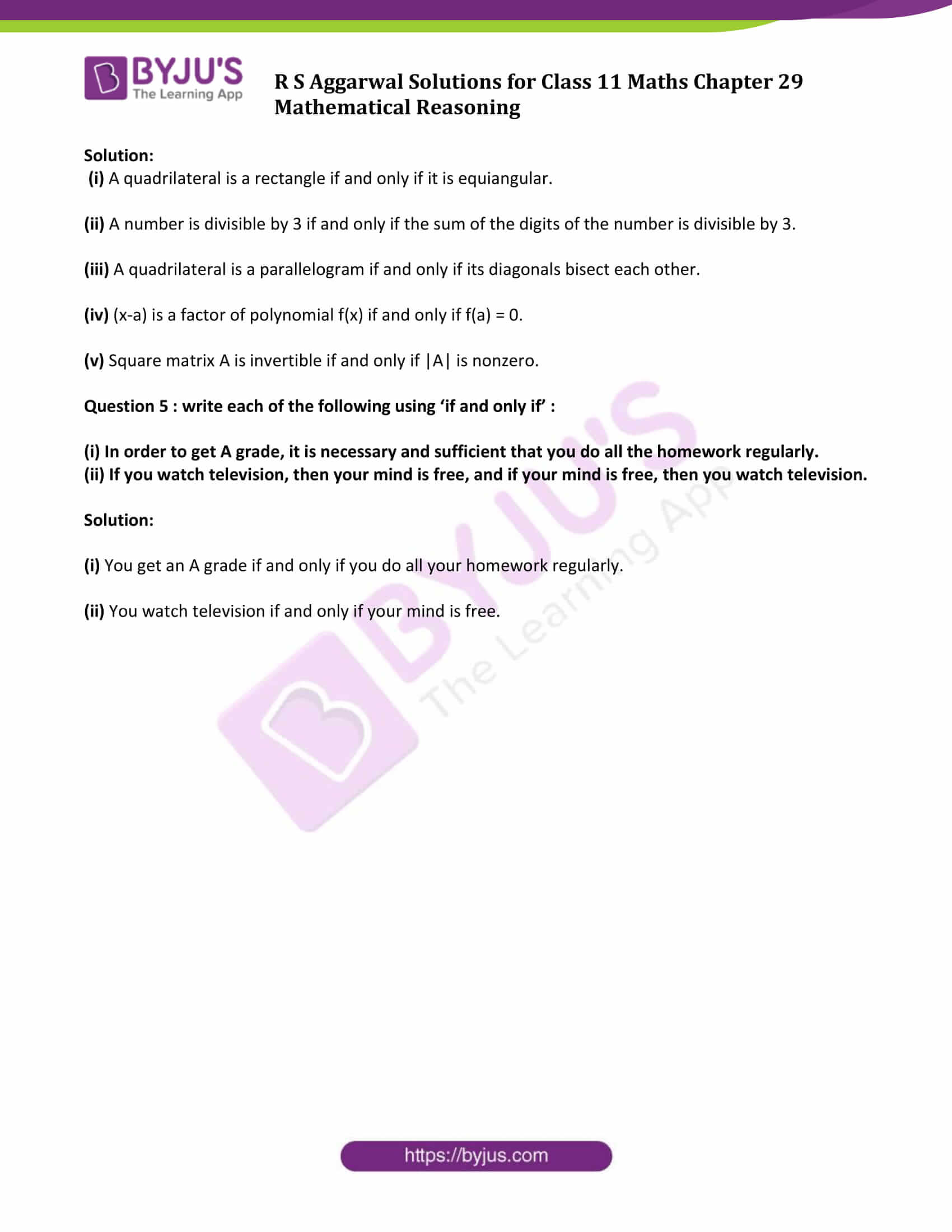# R S Aggarwal Solutions for Class 11 Maths Chapter 29 Mathematical Reasoning Exercise 29C

The R S Aggarwal Class 11 solutions for the chapter Mathematical Reasoning exercise 29C is given here. This exercise is based on conditional and biconditional statements. Conditional statements, are two statements connected by “if…then”. Whereas biconditional statements, are two simple sentences connected by ‘if and only if”. R S Aggarwal class 11 exercise 29C pdf contains solution of all the questions listed in this exercise.

## Download PDF of R S Aggarwal Solutions for Class 11 Maths Chapter 29 Mathematical Reasoning Exercise 29C### Access Answers to Maths R S Aggarwal Class 11 Chapter 29 Mathematical Reasoning Exercise 29C Page number 911

Question 1: Rewrite the following statement in five different ways conveying the same meaning.

If a given number is a multiple of 6, then it is a multiple of 3.

Solution:

(i) A given number is a multiple of 6, it implies that it is a multiple of 3 as well.

(ii) For a given number to be a multiple of 6, it is necessary that it is a multiple of 3.

(iii) A given number is a multiple of 6 only if it is a multiple of 3.

(iv) If the given number in not a multiple of 3, then it is not a multiple of 6.

(v) For a given number to be a multiple of 3, it is sufficient that the number is multiple of 6.

Question 2: Write each of the following statements in the form ‘if …. then’ :

(i) A rhombus is a square only if each of its angles measures 900.

(ii) When a number is a multiple of 9, it is necessarily a multiple of 3.

(iii) You get a job implies that your credentials are good.

(iv) Atmospheric humidity increase only if it rains.

(v) If a number is not a multiple of 3, then it is not a multiple of 6.

Solution:

(i) If each of the angles of a rhombus measures 90°, then the rhombus is a square.

(ii) If a number is a multiple of 9, then the number is multiple of 3.

(iii) If you get a job, then your credentials are good.

(iv) If it rains, then the atmospheric humidity increases.

(v) If a number is a multiple of 6, then it is a multiple of 3.

Question 3: Write the converse and contrapositive of each of the following :

(i) If x is a prime number, then x is odd.

(ii) If a positive integer n is divisible by 9, then the sum of its digits is divisible by 9.

(iii) If the two lines are parallel, then they do not intersect in the same plane.

(iv) If the diagonal of a quadrilateral bisect each other, then it is a parallelogram.

(v) If A and B are subsets of X such that A ⊆ B, then (X – B) ⊆ (X – A)

(vi) If f(2) = 0, then f(x) is divisible by (x – 2).

(vii) If you were born in India, then you are a citizen of India.

(viii) If it rains, then I stay at home.

Solution:

(i) Converse: If x is an odd number, then it is a prime.

Contrapositive: If x is not an odd number, then it is not a prime.

(ii) Converse: If x is an odd number, then it is a prime.

Contrapositive: If the sum of the digits of a positive integer n is not divisible by 9, then n is not divisible by 9.

(iii) Converse: If the two lines do not intersect in the same plane, then they are not parallel.

Contrapositive: If two lines intersect in the same plane, then they are not parallel.

(iv) Converse: If a quadrilateral is a parallelogram, then its diagonals bisect each other.

Contrapositive: If the quadrilateral is not a parallelogram, then its diagonals do not bisect each other.

(v) Converse: If A and B are subsets of X such that (X – B) ⊆ (X – A), then A ⊆ B.

Contrapositive: If A and B are subsets of X such that (X – B) is not a subset of (X – A), then A is not a subset of B.

(vi) Converse: If f(x) is divisible by (x-2), then f(2) = 0.

Contrapositive: If f(x) is not divisible by (x-2), then f(2) ≠ 0.

(vii) Converse: If you are a citizen of India, then you were born in India.

Contrapositive: If you are not a citizen of India, then you were not born in India.

(viii) Converse: If I stay at home, then it rains.

Contrapositive: If I do not stay at home, then it does not rain.

Question 4: Given below are some pairs of statements. Combine each pair using if and only if:

(i) p : If a quadrilateral is equiangular, then it is a rectangle.

q : If a quadrilateral is a rectangle, then it is equiangular.

(ii) p : If the sum of the digits of a number is divisible by 3, then the number is divisible by 3.

q : If a number is divisible by 3, then the sum of its digits is divisible by 3.

(iii) p : A quadrilateral is a parallelogram if its diagonals bisect each other.

q : If the diagonals of a quadrilateral bisect each other, then it is a parallelogram.

(iv) p : If f(a) = 0, then (x – a) is a factor of polynomial f(x).

q : If (x – a) is a factor of polynomial f(x), then f(a) = 0.

(v) p : If a square matrix A is invertible, then |A| is nonzero.

q : If A is a square matrix such that |A| is nonzero, then A is invertible.

Solution:

(i) A quadrilateral is a rectangle if and only if it is equiangular.

(ii) A number is divisible by 3 if and only if the sum of the digits of the number is divisible by 3.

(iii) A quadrilateral is a parallelogram if and only if its diagonals bisect each other.

(iv) (x-a) is a factor of polynomial f(x) if and only if f(a) = 0.

(v) Square matrix A is invertible if and only if |A| is nonzero.

Question 5 : write each of the following using ‘if and only if’ :

(i) In order to get A grade, it is necessary and sufficient that you do all the homework regularly.

(ii) If you watch television, then your mind is free, and if your mind is free, then you watch television.

Solution:

(i) You get an A grade if and only if you do all your homework regularly.

(ii) You watch television if and only if your mind is free.

## Access other exercise solutions of Class 11 Maths Chapter 29 Mathematical Reasoning

Exercise 29A Solutions

Exercise 29B Solutions

Exercise 29D Solutions

## R S Aggarwal Solutions for Class 11 Maths Chapter 29 Exercise 29C

Class 11 Maths Chapter 29 Mathematical Reasoning Exercise 29C is based on the following topics:

• Conditional and Biconditional statements
• Converse and contrapositive of statements
• Biconditional statement
• Various cases showing various conditions